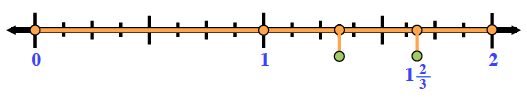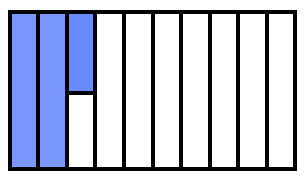### Home > MC1 > Chapter 6 > Lesson 6.2.4 > Problem6-85

6-85.

Represent each of the following numbers two ways: by drawing an area model and by using a number line.

1. $1\frac { 2 } { 3 }$

• Use the number line below to help you draw a diagram.1. $125\%$